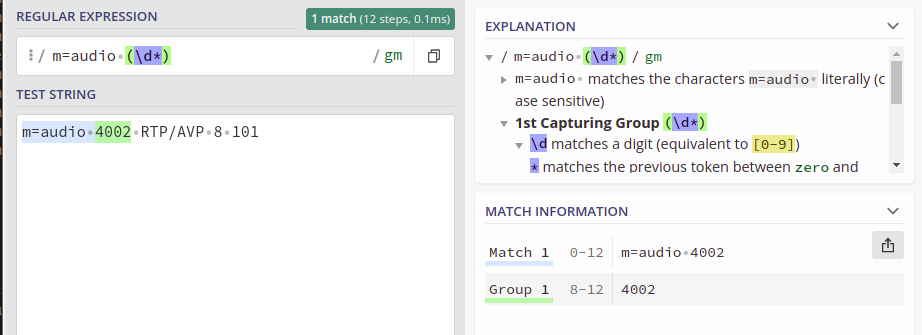# Kamailio Bytes – Extracting SDP Parameters with Kamailio

So the other day I needed to extract the IP and Port parameters from an SDP body – Not the whole line mind, but the values themselves.

As with so many things in Kamailio, there’s a lot of ways to achieve an outcome, but here’s how I approached this problem.

Using the SDPops module we can get a particular line in the SDP, for example, we can get the media line with:

``````#Get SDP line starting with m= and put it into AVP \$avp(mline)
sdp_get_line_startswith("\$avp(mline)", "m=")
#Print value of \$avp(mline)
xlog("m-line: \$avp(mline)\n");``````

This gets us the line, but now we need to extract the data, in the example from the screenshot the M line has the value:

`m=audio 4002 RTP/AVP 8 101`

But we only want the port from the M line.

This is where I’ve used the Kamailio Dialplan module and regex to extract the port from this line.

With a fairly simple regex pattern, we can get a group match for the Port from the m= line.

So I took this regular expression, and put it into the Kamailio Dialplan database with dialplan ID 400 for this example:

`INSERT INTO `dialplan` VALUES (4,400,10,1,'m=audio (\\d*)',0,'m=audio (\\d*)','\\1','SDP M Port Stripper');`

Now using Dialplan ID 400 we can translate an inputted m= SDP line, and get back the port used, so let’s put that into practice:

``````        if(sdp_get_line_startswith("\$avp(mline)", "m=")) {
xlog("m-line: \$avp(mline)\n");
xlog("raw: \$avp(mline)");
xlog("Extracting Port from Media Line");
dp_translate("400", "\$avp(mline)/\$avp(m_port_b_leg)");
xlog("Translated m_port_b_leg is: \$avp(m_port_b_leg)");
}``````

Now we have an AVP called \$avp(m_port_b_leg) which contains the RTP Port from the SDP.

Now we’ve got a few other values we might want to get, such as the IP the RTP is to go to, etc, we can extract this in the same way, with Dialplans and store them as AVPs:

``````        #Print current SDP Values and store as Vars
if(sdp_get_line_startswith("\$avp(mline)", "m=")) {
xlog("m-line: \$avp(mline)\n");
xlog("raw: \$avp(mline)");
xlog("Extracting Port from Media Line");
dp_translate("400", "\$avp(mline)/\$avp(m_port_b_leg)");
xlog("Translated m_port_b_leg is: \$avp(m_port_b_leg)");
}

if(sdp_get_line_startswith("\$avp(oline)", "o=")) {
xlog("o-line: \$avp(oline)\n");
dp_translate("401", "\$avp(oline)/\$avp(o_line_port_1)");
xlog("O Line Port 1: \$avp(o_line_port_1)");
dp_translate("402", "\$avp(oline)/\$avp(o_line_port_2)");
xlog("O Line Port 2: \$avp(o_line_port_2)");
dp_translate("403", "\$avp(oline)/\$avp(o_ip_b_leg)");
xlog("O IP: \$avp(o_ip_b_leg)");
}``````

And all the Regex you’ll need:

```INSERT INTO `dialplan` VALUES
(4,400,10,1,'m=audio (\\d*)',0,'m=audio (\\d*)','\\1','SDP M Port Stripper'),
(5,401,10,1,'o=[^ ]* (\\d*) (\\d*) IN IP4 (\\d*.d*.\\d*.\\d*)',0,'o=[^ ]* (\\d*) (\\d*) IN IP4 (\\d*.d*.\\d*.\\d*)','\\1','O Port 1'),
(6,402,10,1,'o=[^ ]* (\\d*) (\\d*) IN IP4 (\\d*.d*.\\d*.\\d*)',0,'o=[^ ]* (\\d*) (\\d*) IN IP4 (\\d*.d*.\\d*.\\d*)','\\2','O Port 2'),
(7,403,10,1,'o=[^ ]* (\\d*) (\\d*) IN IP4 (\\d*.d*.\\d*.\\d*)',0,'o=[^ ]* (\\d*) (\\d*) IN IP4 (\\d*[.]\\d*[.]\\d*[.]\\d*)','\\3','O IP');

```